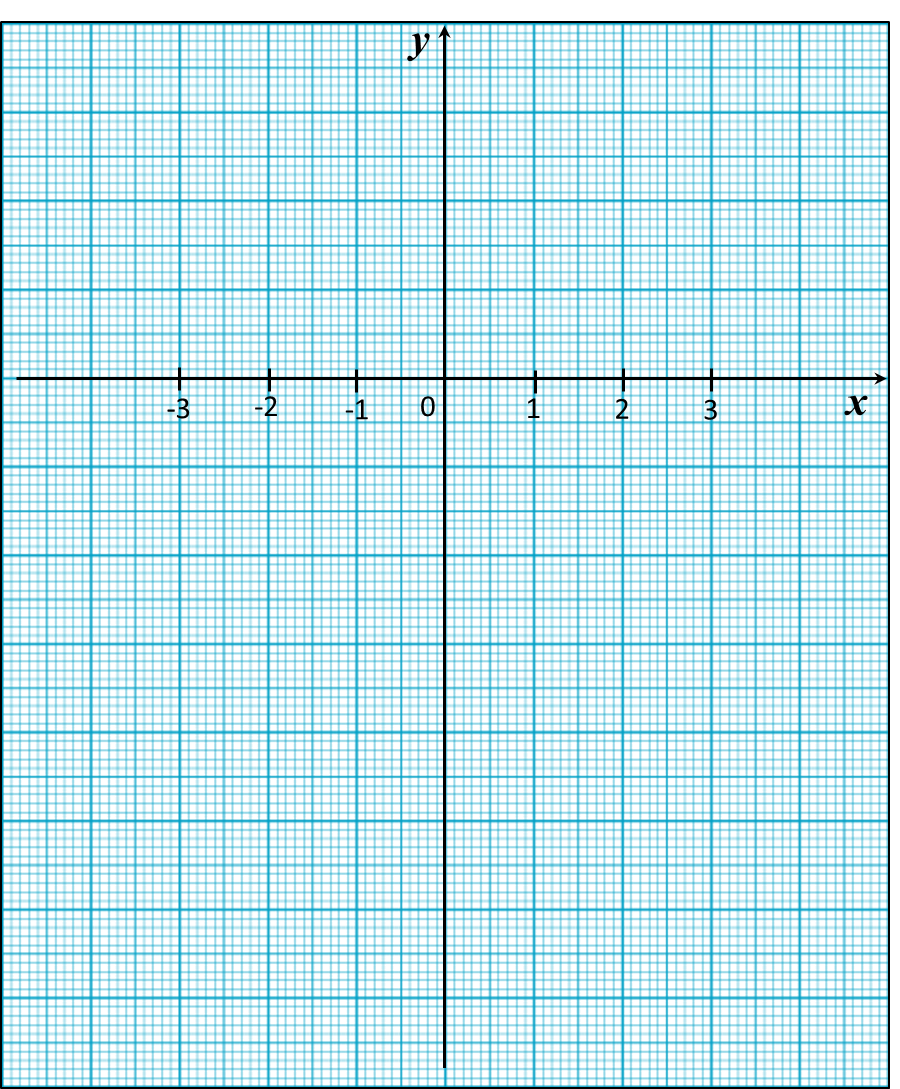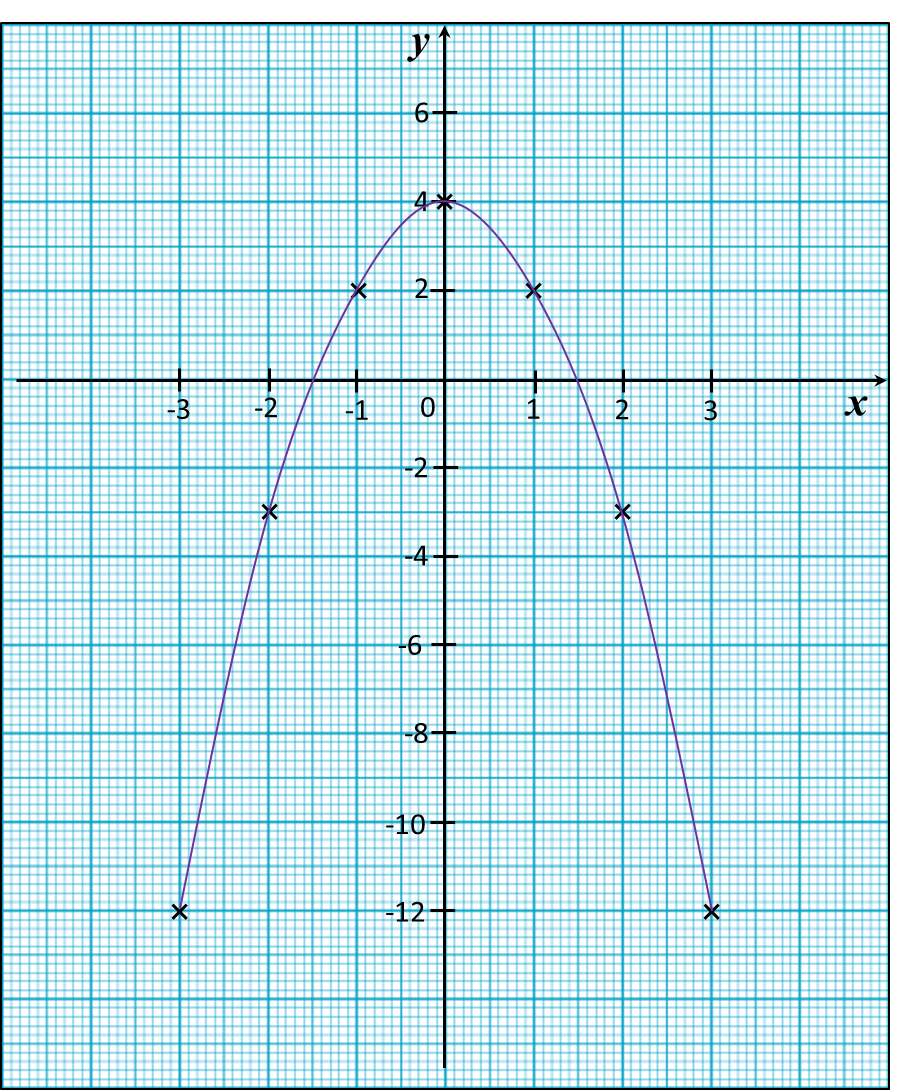# 8.2.4 Graphs of Functions, PT3 Focus Practice

Question 8:
Use graph paper to answer this question.
Table below shows the values of two variables, x and y, of a function.The x-axis and the y-axis are provided on the graph paper on the answer space.

(a) By using a scale of 2 cm to 2 units, complete and label the y-axis.
(b) Based on the table above, plot the points on the graph paper.
(c) Hence, draw the graph of the function.